Related Articles

# Python | Pandas dataframe.reindex()

• Last Updated : 22 Nov, 2018

Python is a great language for doing data analysis, primarily because of the fantastic ecosystem of data-centric python packages. Pandas is one of those packages and makes importing and analyzing data much easier.

Pandas` dataframe.reindex()` function conform DataFrame to new index with optional filling logic, placing NA/NaN in locations having no value in the previous index. A new object is produced unless the new index is equivalent to the current one and copy=False

Syntax: DataFrame.reindex(labels=None, index=None, columns=None, axis=None, method=None, copy=True, level=None, fill_value=nan, limit=None, tolerance=None)

Parameters :
labels : New labels/index to conform the axis specified by ‘axis’ to.
index, columns : New labels / index to conform to. Preferably an Index object to avoid duplicating data
axis : Axis to target. Can be either the axis name (‘index’, ‘columns’) or number (0, 1).
method : {None, ‘backfill’/’bfill’, ‘pad’/’ffill’, ‘nearest’}, optional
copy : Return a new object, even if the passed indexes are the same
level : Broadcast across a level, matching Index values on the passed MultiIndex level
fill_value : Fill existing missing (NaN) values, and any new element needed for successful DataFrame alignment, with this value before computation. If data in both corresponding DataFrame locations is missing the result will be missing.
limit : Maximum number of consecutive elements to forward or backward fill
tolerance : Maximum distance between original and new labels for inexact matches. The values of the index at the matching locations most satisfy the equation abs(index[indexer] – target) <= tolerance.

Returns : reindexed : DataFrame

Example #1: Use `reindex()` function to reindex the dataframe. By default values in the new index that do not have corresponding records in the dataframe are assigned NaN.
Note : We can fill in the missing values by passing a value to the keyword fill_value.

 `# importing pandas as pd``import` `pandas as pd`` ` `# Creating the dataframe ``df ``=` `pd.DataFrame({``"A"``:[``1``, ``5``, ``3``, ``4``, ``2``],``                   ``"B"``:[``3``, ``2``, ``4``, ``3``, ``4``],``                   ``"C"``:[``2``, ``2``, ``7``, ``3``, ``4``],``                   ``"D"``:[``4``, ``3``, ``6``, ``12``, ``7``]},``                   ``index ``=``[``"first"``, ``"second"``, ``"third"``, ``"fourth"``, ``"fifth"``])`` ` `# Print the dataframe``df`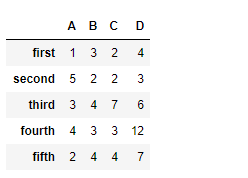Let’s use the `dataframe.reindex()` function to reindex the dataframe

 `# reindexing with new index values``df.reindex([``"first"``, ``"dues"``, ``"trois"``, ``"fourth"``, ``"fifth"``])`

Output :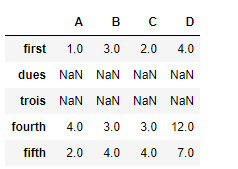Notice the output, new indexes are populated with `NaN` values, we can fill in the missing values using the parameter, fill_value

 `# filling the missing values by 100``df.reindex([``"first"``, ``"dues"``, ``"trois"``, ``"fourth"``, ``"fifth"``], fill_value ``=` `100``)`

Output :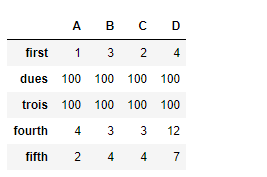Example #2: Use `reindex()` function to reindex the column axis

 `# importing pandas as pd``import` `pandas as pd`` ` `# Creating the first dataframe ``df1 ``=` `pd.DataFrame({``"A"``:[``1``, ``5``, ``3``, ``4``, ``2``],``                    ``"B"``:[``3``, ``2``, ``4``, ``3``, ``4``],``                    ``"C"``:[``2``, ``2``, ``7``, ``3``, ``4``],``                    ``"D"``:[``4``, ``3``, ``6``, ``12``, ``7``]})`` ` `# reindexing the column axis with``# old and new index values``df.reindex(columns ``=``[``"A"``, ``"B"``, ``"D"``, ``"E"``])`

Output :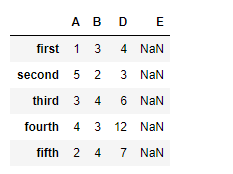Notice, we have `NaN` values in the new columns after reindexing, we can take care of the missing values at the time of reindexing. By passing an argument `fill_value `to the function.

 `# reindex the columns``# fill the missing values by 25``df.reindex(columns ``=``[``"A"``, ``"B"``, ``"D"``, ``"E"``], fill_value ``=` `25``)`

Output :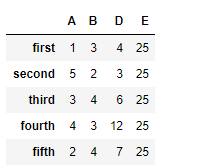Attention geek! Strengthen your foundations with the Python Programming Foundation Course and learn the basics.

To begin with, your interview preparations Enhance your Data Structures concepts with the Python DS Course. And to begin with your Machine Learning Journey, join the Machine Learning – Basic Level Course

My Personal Notes arrow_drop_up